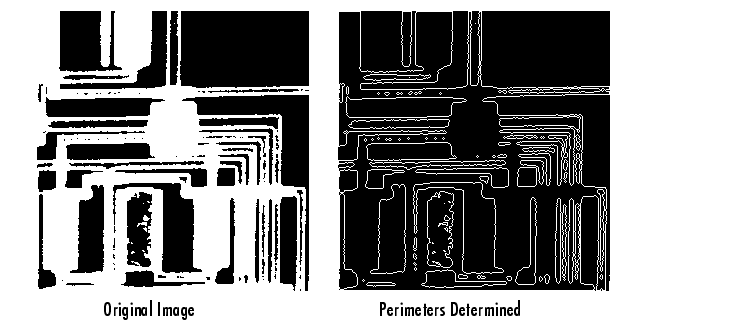Image Processing Toolbox User's GuideDilation- and Erosion-Based Functions

This section describes two common image processing operations that are based on dilation and erosion:

This table lists other functions in the toolbox that perform common morphological operations that are based on dilation and erosion. For more information about these functions, see their reference pages.

 Function Morphological Definition `bwhitmiss` Logical `AND` of an image, eroded with one structuring element, and the image's complement, eroded with a second structuring element. `imbothat` Subtracts the original image from a morphologically closed version of the image. Can be used to find intensity troughs in an image. `imclose` Dilates an image and then erodes the dilated image using the same structuring element for both operations. `imopen` Erodes an image and then dilates the eroded image using the same structuring element for both operations. `imtophat` Subtracts a morphologically opened image from the original image. Can be used to enhance contrast in an image.

Skeletonization

To reduce all objects in an image to lines, without changing the essential structure of the image, use the `bwmorph` function. This process is known as skeletonization.

• ```BW1 = imread('circbw.tif');
BW2 = bwmorph(BW1,'skel',Inf);
imshow(BW1)
figure, imshow(BW2)```

Perimeter Determination

The `bwperim` function determines the perimeter pixels of the objects in a binary image. A pixel is considered a perimeter pixel if it satisfies both of these criteria:

• The pixel is `on`.
• One (or more) of the pixels in its neighborhood is `off`.

For example, this code finds the perimeter pixels in a binary image of a circuit board.

• ```BW1 = imread('circbw.tif');
BW2 = bwperim(BW1);
imshow(BW1)
figure, imshow(BW2)```Combining Dilation and Erosion Morphological Reconstruction